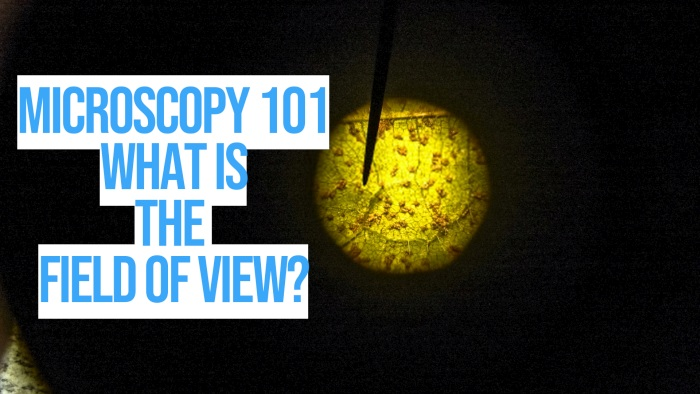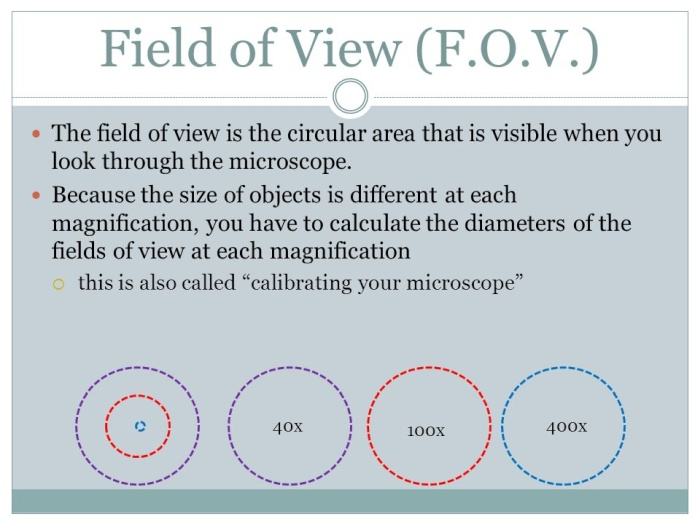# Microscopy 101: Field of View

The field of microscopy can be fun and exciting, as you get to explore many different possibilities in the world around you. But, to fully understand how microscopy works, it’s important to learn about its basic principles and underlying concepts.

This includes having an idea about how light refraction works, understanding the concepts of magnification and resolution, and many other principles, including knowing what the field of view is, and how to calculate it.

Contents

## What is the field of view?

Field of view is defined as the extent of the observable world at any given point in time. It’s the range of visibility, typically measured as an angle, that the eye or an optical instrument is capable of.

When it comes to the human eye, field of view is often referred to as the visual field, and it’s usually a horizontal arc measuring a little over 210 degrees, meaning, we can see everything in front of us as long as it is within this range.

Different animals and optical instruments also have different fields of view. Below is an explanation of microscopy field of view, how it’s calculated, and the different factors that affect it.

## What is the field of view in microscopy?

In a microscope, the microscopy field of view is the diameter of the viewing field measured at an intermediate plane of angle. To put it simply, it’s the diameter of the circular area you see when you look through the eyepiece of the microscope.

In the case of optical microscopes, such as light microscopes, the field of view is determined by the diameter of the opening of the eyepiece field diaphragm, which can either be in between the objective lens and the ocular lens, or before the two lenses.

And, it’s also dependent on the magnification of the objective lens, or more accurately, the total compounded magnification of the objective and ocular lenses.

## What determines the microscopy field of view?

As mentioned above, the field of view is determined by the diameter of the diaphragm, and the magnification of the lenses. You can usually find these numbers imprinted on the side of the microscope’s eyepiece.The first number, ending with an X, is the magnification, while the second number is the diameter. This is called the field of view number, or simply field number, and it’s expressed in millimeters.

The maximum field diameter typically falls within a range of 18 to 28 millimeters (or more), depending on how advanced the objective lens is, such as whether the lens is a special type of a flat field objective.

## How do you calculate the microscope’s field of view?

Based on the definition of the microscopy field of view above, we can then infer that the viewing field size of the specimen plane as the field number divided by the objective lens’ magnification, or Field size = field number/ objective magnification.

The field number of the microscope eyepiece is typically restricted by the size of its field diaphragm and its magnification, but this can be somewhat affected if there are any auxiliary lenses with their own magnification placed in between the objective and ocular lenses.

If this is the case, the compounded magnification of the objective and auxiliary lens should be calculated by multiplying the two together, and this total magnification is what should be used in calculating the field size.Example

For example, if the maximum field diameter of the diaphragm is 20 millimeters, and the microscope has two lenses with a magnification each of 20x and 10x respectively, we can calculate the viewing field size as 20 millimeters divided by 200, equating to 0.1 millimeter.

Alternatively, if the total magnification is only 20x, then the field size becomes 1 millimeter, which means you can see a bigger portion of the specimen. But, you can’t see as many minute details, since the magnification is low.

This is why the field of view of a simple microscope is as big as a few centimeters wide, while the field size of a transmission electron microscope is only a single nanometer to a few picometers.

## How does magnification affect the field of view?

From the example above, we can conclude that the microscope’s magnification conversely affects its field of view- the more times the specimen is magnified, the less of it you can see. Or at least, less in terms of area size, but more in terms of detail.

To give you an idea, an ocular lens or eyepiece with a magnification of 5x normally has a 20 millimeter field number, while a 10x magnification eyepiece has a somewhat smaller field number of 16 to 18 millimeters. The difference becomes noticeable as the magnification increases to a hundred or more.

## Why is the field of view important?

The microscopy field of view is what determines how much of the specimen we can see. Understanding how it works means you can set your expectations on what you can view under the microscope as you manipulate the magnification power.

To help you visualize, let’s say you are looking at an insect specimen. If you want to be able to see the entire insect under the microscope, you need to use a low power lens.

But, if you want to “zoom in” on a certain part such as the wing, you need to increase the magnification, and move the specimen slide slightly, until the part you want to see is centered on the viewing field.

## Summary

The microscopy field of view is the total visible area of the specimen plane, which is determined by the field number or the diameter of the diaphragm, and the magnification of the lens. This viewing field size is conversely affected by the magnification level of the microscope.

While the best way to increase the field of view is by lowering the magnification of the microscope, this can be inefficient and counter-productive. This is why more sophisticated lenses have been developed to offer a wider viewing plane without sacrificing magnification.

### 1 thought on “Microscopy 101: Field of View”

1.Thanks .I want to join your page because I’m a science student.

Scroll to Top
Scroll to Top